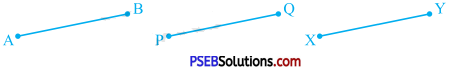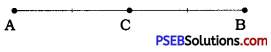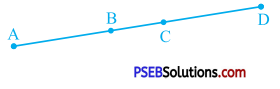# PSEB 9th Class Maths Solutions Chapter 5 Introduction to Euclid’s Geometry Ex 5.1

Punjab State Board PSEB 9th Class Maths Book Solutions Chapter 5 Introduction to Euclid’s Geometry Ex 5.1 Textbook Exercise Questions and Answers.

## PSEB Solutions for Class 9 Maths Chapter 5 Introduction to Euclid’s Geometry Ex 5.1

Question 1.
Which of the following statements are true and which are false? Give reasons for your answers:
(i) Only one line can pass through a single point.
lines or perpendicular lines where two lines pass through their point of intersection which is always unique.

(ii) There are an infinite number of lines which pass through two distinct points.
The given statement is false because if it is so. the distance between two points can never be defined. Also, it contradicts postulate 1.(iii) A terminated line can be produced indefinitely on both the sides.
The given statement is true according to postulate 2.

(iv) If two circles are equal, then their radii are equal.
The given statement is true. if two circles are equal. their circumferences as well as their centres will coincide when placed on each other. Now, radius is the distance between centre of a circle and any point on the circle. Hence, their radii are also equal.

(v) In the given figure, if AB = PQ and PQ = XY, then AB = XY.The given statement is true according to axiom 1. Here. AB and XY are equal to the same thing PQ. Hence, AB and XY are equal, i.e., AB = XY.Question 2.
Give a definition for each of the following terms. Are there other terms that need to be defined first? What are they, and how might you define them?
(i) Parallel lines
Parallel lines: Two lines which lie in the same plane and do not intersect anywhere are called parallel lines.

(ii) Perpendicular lines
Perpendicular lines: Two lines intersecting each other at right angles are called perpendicular lines.

(iii) Line segment
Line segment: Line segment is a part of a line and having two end-points. In other words, line segment is a terminated line.Radius of a circle: Any line segment joining the centre of a circle to any point on its circumference is called a radius of a circle.
Note : The length of the radius of a circle is also referred as radius of the circle.

(v) Square
Square: A quadrilateral with all the four sides equal and all the four angles equal (90° each) is called a square.
There are many other terms like line, plane, right angle, centre of a circle, circumference and quadrilateral that need to be defined first. Among them, line and plane cannot be defined so they are taken as undefined terms. Other four terms can be defined as below:

• Right angle: An angle of 90° is called a right angle.
• Centre of a circle: A point in the plane of a circle which is equidistant from all the points of the circle is called the centre of the circle.
• Circumference: The length of the boundary of a circle is called its circumference.
• Quadrilateral: A closed plane figure having four sides and four angles is called a quadrilateral.Question 3.
Consider two ‘postulates’ given below:
(i) Given any two distinc’t points A and B, there exists a third point C which is in between A and B.
(ii) There exist at least three points that are not on the same line.
Do these postulates contain any undefined terms? Are these postulates consistent? Do they follow from Euclid’s postulates? Explain.
Yes. These postulates contain undefined terms like point and line.
Yes. These postulates are consistent.
Postulate (i) states that given two distinct points A and B, there is a point C on line AB which lies between A and B.
Postulate (ii) states that given two distinct points A and B. we have atleast one point C which does not lie on line AB.
These postulates do not follow from Euclid’s postulates. They follow from axiom 5.1.

Question 4.
If a point C lies between two points A and B such that AC = BC. then prove that AC = $$\frac{1}{2}$$AB. Explain by drawing the figure.
AC = BC
∴ AC + AC = BC + AC
[Equals are added to equals and axiom 2]
∴ 2AC = AB [BC + AC coincides with AB and axiom 4]
∴ $$\frac{1}{2}$$(2AC) = $$\frac{1}{2}$$AB lAxiom 7]
∴ AC = $$\frac{1}{2}$$ABQuestion 5.
In Q. 4, point C is called a midpoint of line segment AB. Prove that every line segment has one and only one midpoint.
From question 4, we get two properties of the midpoint C of line segment AB. (i) C is a point lying on line segment AB between A and 8. (ii) C is equidistant from A and B.

If possible, suppose point D other than point C is also a midpoint of line segment AB. Then, according to question 4, we have AD = $$\frac{1}{2}$$ AB.
Moreover. AC = $$\frac{1}{2}$$ AB is proved in Q. 4.
∴ AD = AC [Axiom 1]
Now, D and C both lie on line segment AB between A and B and at the same distance from A. This proves that D and C are the same points. Hence, every line segment has one and only one midpoint.

Question 6.
In the given figure, if AC = BD, then prove that AB = CD.